# MA.912.LT.4.2Export Print
Determine truth values of simple and compound statements using truth tables.
General Information
Subject Area: Mathematics (B.E.S.T.)
Strand: Logic and Discrete Theory
Status: State Board Approved

## Benchmark Instructional Guide

### Vertical Alignment

Previous Benchmarks

Next Benchmarks

### Purpose and Instructional Strategies

In Math for College Liberal Arts, students determine truth values of simple and compound statements using truth tables. In other courses, students refine their knowledge and skills regarding logic and set theory.
• Building on MA.912.LT.4.1, instruction includes understanding that logic is concerned with propositional statements that are either true or false. Every propositional statement in logic has exactly one of the two truth values (either true or false, but not both or neither).
• Building on MA.912.LT.4.1, students determine when compound propositional statements involving negation, conjunction, disjunction, conditional, and biconditional, are true and when they are false. The truth-value relationships among several statements may be represented by a diagram called a truth table.
• Truth table is a table showing the resulting truth value of a compound statement for all the possible truth values for the simple statements.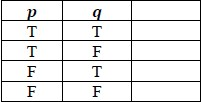• Instruction includes the formal definition of the basic truth tables for negation, conjunction, disjunction, conditional, and biconditional.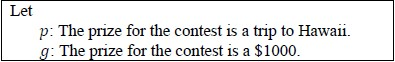• Negation (~) changes the truth value of a statement; a true statement becomes false, and a false statement becomes true. This can be expressed in a truth table, where T represents true and F represents false.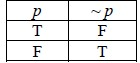• For example, if the statement p:The prize for the contest is a trip to Hawaii is true, then the negation of this statement ~ p: The prize for the contest is NOT a trip to Hawaii is false.
• Conjunction (∧) is true only when both p and q are true.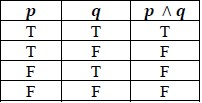• For example, the statement pq: The prize for the contest is a trip to Hawaii AND \$1000 is true only when both statement p and statement q are true; otherwise, the conjunction is false.
• Disjunction (∨) is false only when both p and q are false.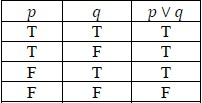• For example, the statement pq: The prize for the contest is a trip to Hawaii OR \$1000 is false only when both statement p and statement q are false; otherwise, the disjunction is true.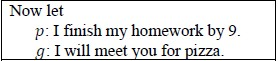• Conditional (→) is false only when the hypothesis (p) is true, but the conclusion (q) is false; this relationship may be thought of as ‘breaking a promise.’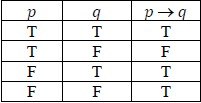• For example, pq: IF I finish my homework by 9, THEN I will meet you for pizza is false only when I do finish my homework by 9, but I do not meet you for pizza.
• Biconditional (↔) is true only when p and q have the same truth value. A biconditional is a conjunction of two conditional statements, (pq) ∧ (qp).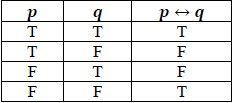• For example, pq: I finish my homework by 9, IF AND ONLY IF I will meet you for pizza. Biconditional can be considered as two conditional statements, pq: If I finish my homework by 9, then I'll meet you for pizza; and qp: If I will meet you for pizza, then I finish my homework by 9. Since the conditional is false only when the hypothesis is true, and the conclusion is false, biconditional is true only when both p and q are true, or when both are false.
• Instruction includes determining the truth value of a given compound statement using a truth table. Each operation is a different column in the truth table (see highlights in the table example below). The order of the operations starts with the inside of the parentheses.
• For example, determine the truth value of the following compound statement: (~p ∧ q) → p. Following the order of operations, first find the negation of p, then the conjunction, and finally the conditional.
• To complete column 3, negate column 1.
• To complete column 4, find the conjunction of column 3 AND column 2.
• Column 5 is an optional copy of column 1 to assist with column 6.
• To complete column 6, find the conditional of column 4 and column 5.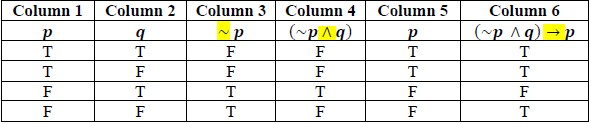### Common Misconceptions or Errors

• Students may assume that in a conditional truth table, when the hypothesis is false, then the conditional is false. To address this, instruction should specify that the conditional is statement is true by default in the case when hypothesis is false, regardless of the truth value of the conclusion.

• Given the statement: If today is Monday, then the unicorns are pink with purple stripes.
• Part A. Is this statement true today?
• Part B. When is this statement false?
• Part C. Create a truth table to confirm your hypotheses in Part A and Part B.

### Instructional Items

Instructional Item 1
• Complete a truth table for (p ∨∼ q) ↔ q.

*The strategies, tasks and items included in the B1G-M are examples and should not be considered comprehensive.

## Related Courses

This benchmark is part of these courses.
7912070: Access Mathematics for Liberal Arts (Specifically in versions: 2014 - 2015, 2015 - 2018, 2018 - 2019, 2019 - 2022, 2022 - 2023, 2023 and beyond (current))
1207350: Mathematics for College Liberal Arts (Specifically in versions: 2022 and beyond (current))
1212300: Discrete Mathematics Honors (Specifically in versions: 2022 and beyond (current))

## Related Access Points

Alternate version of this benchmark for students with significant cognitive disabilities.

## Related Resources

Vetted resources educators can use to teach the concepts and skills in this benchmark.

## Student Resources

Vetted resources students can use to learn the concepts and skills in this benchmark.

## Parent Resources

Vetted resources caregivers can use to help students learn the concepts and skills in this benchmark.